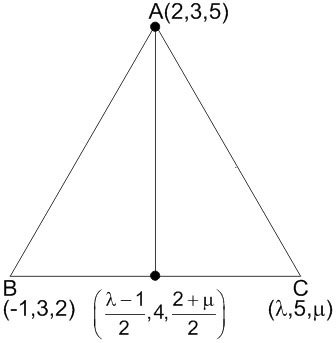# Let A (2, 3 ,5),  B (-1, 3, 2) and Cbe the vertices of aABC. If the median through A is equally inclined to the coordinate axes, then : Option 1)Option 2)Option 3)Option 4)P Prateek Shrivastava

As we learnt in

Direction Ratios -

(i)     if a,b,c are direction ratios then direction cosines will be

(ii)        Direction ratios of line joining two given points

is given by

(iii)    If  be a vector with direction cosines l, m, n then

-DRS of median =

All are same,

Thus,Option 1)This opttion is incorrect

Option 2)This opttion is incorrect

Option 3)This opttion is correct

Option 4)This opttion is incorrect

Exams
Articles
Questions